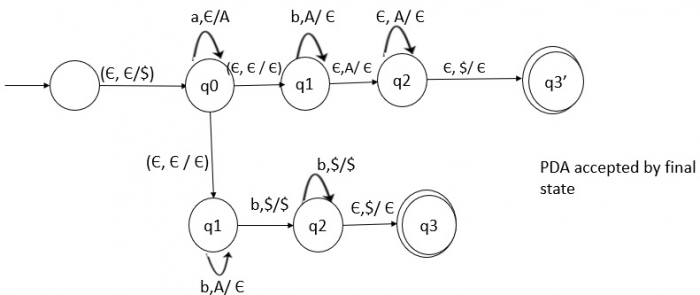# Design a PDA which recognizes the language

## Problem

Generate the push down automata (PDA) that recognizes the language E={aibj| i is not equal to j and I is not equal to 2j}.

## Solution

Consider the two languages as given below −

L1={aibj|i,j>=0 and i>2j}L2={aibj|i,j>=0 and i<2j}

Convince yourself that L=L1UL2

In L1, the number of a's are more than double of b's so L1 as follows −

S1->aA

A->aaAb|aA|epsilon

In L2, the number of a's are less than double the number of b's

So the CFG for L2 becomes as follows −

S2->Bb|aBb

B->Bb|aBb|aaBb|epsilon

S->S1|S2

L1: {aibj:i>2j}L2:{aibj: i<2j}